### Home > CALC > Chapter 10 > Lesson 10.2.1 > Problem10-89

10-89.
1. Determine if the following series converge or diverge. State the test you used. Homework Help ✎

1.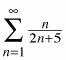2.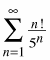3.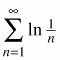4.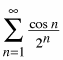5.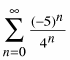6.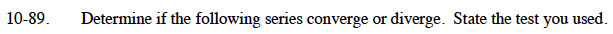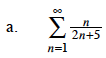$\text{Is }\lim_{n\to\infty}\frac{n}{2n+5}=0?$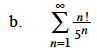Use the Ratio Test.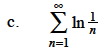$\text{Is }\lim_{n\to\infty}\ln\Big(\frac{1}{n}\Big)=0?$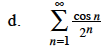$\left|\cos(n)\right|\le1$

$\frac{\cos(n)}{2^n}\le\frac{1}{2^n}$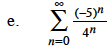This is a geometric series. What is the value of r?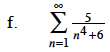Use the Limit Comparison Test with 1/n4.